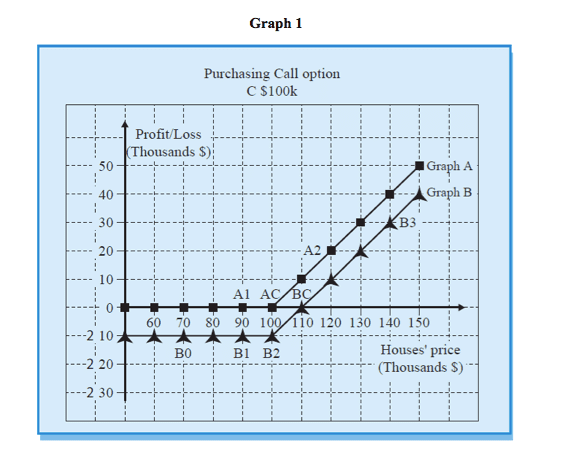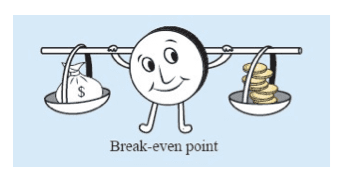# Buying a Call Option – Graphic Presentation

## Graphic Presentation of Profit (or Loss) from the Option on date B

The following graph shows the profit (or loss) on date B from purchasing option Call \$100K on houses.

We paid \$10K for the option.

There are 2 graphs:

Graph A – profit line for the option (gross profit).

Graph B – profit line for the transaction (net profit).Graph A shows what our profit will be on the option on date B, for any price level on that date in the housing market.

For example at Point A2 shows a profit of \$20K, if the price of the houses in \$120K.

Point A1 shows that we make no profit at all (0 profit), if the price of the houses in \$90K

Graph A does not drop below the \$0 point, which means that we cannot lose on an option we received free of charge.

Graph A is presented only for sake of convenience. Graph B is the relevant graph, since it relates to the profit or loss on the transaction.

Graph B

Shows us the profit or loss on the transaction, for the same level of prices. (Graph B is lower than Graph A by the amount of the premium – \$10K).

For example: Point B3 shows a transaction profit of \$30K, if house prices on date B are \$140K. Point B1 shows that we will lose \$10K on the transaction, if house prices on date B are \$90K.

Also at Point B0 (house prices at \$70K), we lose only \$10K – in other words we cannot lose more than \$10K, which is the amount of the premium.

From Graph B it can be seen that the profit from the transaction increases as the price of houses goes up. (Graph A also presents the same picture). From Point B2  rightwards, each increase of \$1K in house prices  contributes us \$1K.

At Point BC we have covered the premium expense, and we are in balance. This point is called the “break-even point”.

For every increase of \$1K in house process, we make a profit of \$1K (ratio of 1:1).

When the price of houses increases from \$110K to \$120K,  we make a profit of \$10K.

Our loss on the transaction is limited to the amount of the premium, as is shown by Graph B leftwards from B2.

Exercising the option

When the price of houses exceeds \$100K, it is worth exercising the option. If we do not exercise the option at a price of \$101K, we lose the whole value of the premium (\$10K). On the contrary, if we exercise it, we lose only \$9K. When the price of houses is less than \$100K, it is not worth exercising the option. Exercising the option will result in an additional loss, over and above the loss of the premium.